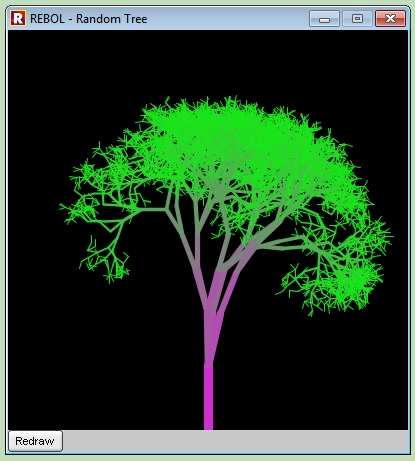Neocities / Hodgepodge / Programming / Rebol2 Recursive Tree
```
(this is just a screenshot; code is below that)(and it'll draw a different tree each time)

The Code (grntree.r):
rebol [title: "Random Tree"]

random/seed now/precise

rnd: func [L H][L + (random 1e9 * (H - L) / 1e9)]
as-tuple: func[A B C][to-tuple reduce [A B C]]

trunk: 64

branch: funct [X Y L A][
Y2: Y - (L * cosine A)
X2: X + (L * sine A)
C: (to-integer (L * (rnd 200 255) / trunk))
append block compose [
line-width (L / 7)
pen (as-tuple C (255 - C) C)
line (as-pair X Y) (as-pair X2 Y2)
]
if L > 8 [
loop (random 3) [
branch X2 Y2 (L * 0.83) (A + ((rnd -1300 1300) / L))
]
]
]

dr: does[block: copy [line-cap rounded] branch 200 399 trunk 0]
dr

view layout [
origin 0x0 space 0x0
b: box black 400x400 effect reduce ['draw block]
btn "Redraw" [dr b/effect: reduce ['draw block] show b]
]```